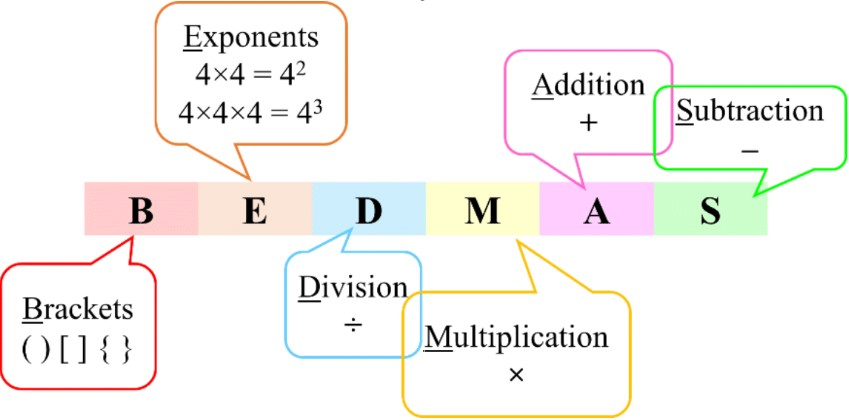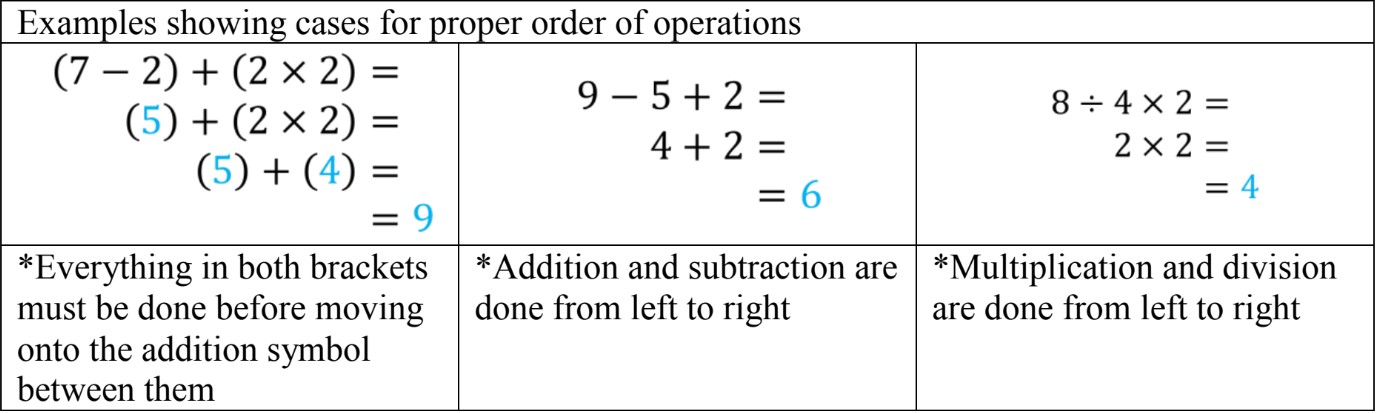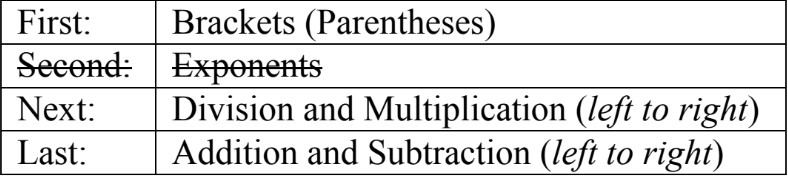# Applications of the four operations (BEDMAS without exponents)#### All in One Place

Everything you need for better grades in university, high school and elementary.#### Learn with Ease

Made in Canada with help for all provincial curriculums, so you can study in confidence.#### Instant and Unlimited Help

0/4
##### Intros
###### Lessons
1. Introduction to Applications of the Four Operations (BEDMAS):
2. Why do we need Order of Operations?
3. BEDMAS and Order of Operations (without exponents)
4. Special case examples: proper order for multiple operations in equations
5. Review on BEDMAS steps
0/11
##### Examples
###### Lessons
Fill in the blanks for the distributive property model.
1. 2 × (2 + 5) = ___
(2 × __) + (2 × __) = ___
2. 4 × (3 + 1) = ___
(4 × __) + (4 × __) = ___
2. Applying BEDMAS (without exponents)
Follow the correct order and solve
1. (3 × 6) + 10 =
2. (8 - 3) × 9 =
3. 1 + 5 × 8 =
4. 12 ÷ 2 - 6 =
5. 30 - 4 × 7 + 3 =
6. 9 × 2 - 20 ÷ 5 =
7. 36 + 4 ÷ 4 - 8 × 2 =
3. Word Problem with Order of Operations (BEDMAS) - 1
Sierra bought 6 bunches of bananas for 3 dollars each and 4 bunches of grapes for 8 dollars each.
• Write an equation to represent the word problem.
• Then, draw brackets around the operation(s) that need to be done first.
• Finally, find the total cost of the fruits Sierra bought.
1. Word Problem with Order of Operations (BEDMAS) - 2
Reilly planned a bake sale to fundraise for his local children's hospital. After the bake sale was done, he counted 120 dollars. The ingredients he bought to make his cupcakes cost him 30 dollars.
His bank wanted to help and said they would match his donation to double his profit.
• How much profit did Reilly make on his own? (sales - expenses)
• Write an equation for the total fundraised amount that will be donated.
• What is the total amount donated to the local children's hospital?
###### Topic Notes

In this lesson, we will learn:

• How to solve equations with multiple different operations in them
• How to follow the proper order of operations using BEDMAS (or PEMDAS)

Notes:

• Order of operations tells us the rules for which math operations to do first, second, third…
• The 4 basic operations are: addition (+), subtraction (-), multiplication (×), and division (÷)

• We CANNOT do operations in any order we want because it will lead to different answers.
• Mathematicians have agreed on the order so that everyone does all the operations in the same order to get the same answer (only one correct answer).

• To remember the order, we can use the acronym BEDMAS• Alternatively, some textbooks also use the acronym PEMDAS instead
• P in PEMDAS stands for parentheses; M and D still stand for Division and Multiplication, but they've switched places from BEDMAS

• If there are more than one of each type of operation, they can all be done in the same step, from left to right, before moving onto the next step.• The steps for Order of Operations (without exponents) is: